# 1st PUC Computer Science Previous Year Question Paper March 2015 (North)

Students can Download 1st PUC Computer Science Previous Year Question Paper March 2015 (North), Karnataka 1st PUC Computer Science Model Question Papers with Answers helps you to revise the complete Karnataka State Board Syllabus and score more marks in your examinations.

## Karnataka 1st PUC Computer Science Previous Year Question Paper March 2015 (North)

Time: 3:15 Hours
Max. Marks: 100

PART – A

I. Answer all the following questions. Each question carries one mark. (10 × 1 = 10)

Question 1.
Who Invented the Pascaline?
French Philosopher Blaise Pascal invented the Pascaline.

Question 2.
What is the use of the Input Unit?
It is used to feed data and instructions into the CPU fed by the user through input devices.

Question 3.
Mention, anyone tool used in the design of the problem?
The flowchart is a tool used in the design of problem solution.Question 4.
Define Constant in C++.
The constant is a data in which meaning remains unchanged through out the program.

Question 5.
Write the purpose of Cin>> Statement.
The purpose of Cin>> statement is to input data values in a program.

Question 6.
Where selection statements are used?
Answer: The selection statements are used in decision making process where more than one alternative task to be selected for execution.

Question 7.
What is the first subscript value of the array in C++?
The first subscript value of the array in C++ is zero (0).Question 8.
What is a User-defined function?
The user-defined functions are declared and defined in the program by the programmer which perform specific activity and give expected result.

Question 9.
Mention the component of font dialog box in Word Processor?
The Font Name, Style, Size, etc., are the components of font dialog box in word processor.

Question 10.
Expand ESS?
The ESS is expanded as “Electronic Spread Sheet”.

PART – B

II. Answer any Five of the following questions. Each question carries two marks: (5 × 2 = 10)

Question 11.
Mention any two features of ENIAC.
The two features of ENIAC first generation computer is High power consumption, High heat generation, and machine language was used to operate. It is mainly used for Calculation and computation, Payroll Processing and Record keeping.

Question 12.
Write the differences between impact and nonimpact?
The differences between impact and nonimpact printers are:

 Impact printer Non-impact printer Produces text and images when tiny wire pins on print head strike the ink ribbon by physically contacting the paper Produces text and graphics on paper without actually striking the paper Low printing speed Reasonably fast Print quality lower in some types High quality of output, capable of printing fine and smooth details Uses ink ribbon Uses ink spray or toner powder Less expensive More expensive

Question 13.
Differentiate Interpreter and Compiler.
The differences between interpreter and compiler are:

 Interpreter Compiler Translates Program line by line Translates a whole program at a time. Remains in RAM After translation Leaves RAM after translation. Suitable for small translations Suitable for long computation Slower access Faster access.Question 14.
Write any two symbols used in the Flowcharts.
The two symbols used in the flowcharts are: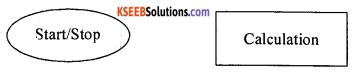Question 15.
Mention any two benefits of OOP.
The two benefits of OOP are

1. Follow bottom-up approach in program design.
2. Functions and data are coupled together.

Data encapsulation and inheritance are the two features of OOP.

Question 16.
Classify various data types in C++.
Data types are classified into built in or basic data types and user defined or derived data types.

Question 17.
Write the purpose of Isalpha() and Toupper() built in function,
1. isalpha() returns a non-zero value if argument is A-Z or a-z letters.
For example, isalpha(‘a’) output: true
isalpha(‘5’) output: false

2. toupper () function converts the lower case alphabet character to upper case.
For example, toupper(‘a’) output: AQuestion 18.
Give the command for Copy and Paste in Word Processor.
The copy command is Ctrl + C and Ctrl + V is for Paste in Word Processor.

PART – C

III. Answer any Five of the following Question. Each question carries three marks: (5 × 3 = 15)

Question 19.
Discuss the characteristics of a computer in detail, (any three)
The main characteristics of a computer are:
Computers are fast in doing calculations. The speed of the computer is measured in terms of million instruction per second (MIPS).
1. Storage Capacity:
Computers come with large amount of memory. They can hold lot of data. Computers can show a particular piece of information from large amount of data in a short time.

2. Diligence:
After doing work for sometime, humans become tired but computers do not become tired. They work continuously. In fact, some computers which control telephone exchanges are never stopped. This is called diligence.

3. Accuracy:
The results that the computers produce are accurate provided data and programs are reliable.

Question 20.
With the help of an example explain 1’s complement and 2’s complements.
1’s complement:
Representing a signed number with 1’s complement is done by changing all the bits that are 1 to 0 and all the bits that are 0 to For example, 1’s complement of 1010100 is 0101011.

2’s complement:
Representing a signed number with 2’s complement is done by adding 1 to the 1’s complement representation of the number. For example, 2’s complement of 1010100 is

• Step 1: 1’s complement of 1010100 is 0101011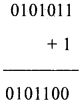Question 21.
Classify any three types of operating system.
The three types of operating systems are:
1. Single user operating sytems:
Single user operating systems allows only one user to share the system resources including the CPU. For example, DOS (disk operating system).

2. Batch operating system:
In a batch processing operating system interaction between the user and processor is limited or there is no interaction at all during the execution of work. Data and programs that need to be processed are bundled and collected as a ‘batch’ and •
executed together. For example, IBM OS/2.

In multi tasking operating system several applications maybe simultaneously loaded and used in the memory. While the processor handles only one application at a particular time and switch between the applications and simultaneously execute each application. For example, Windows operating system.

4. Multi user operating system:
This multi user operating system allows multiple users to simultaneously use the system, the processor splits its resources and handles one user at a time. For example, UNIX.

Question 22.
Draw the flowchart to find area and circumference of a circle.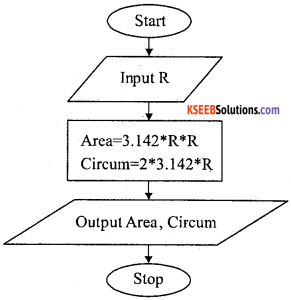Question 23.
Mention the three characteristics of C++ programming language.
Object oriented programming, bottom-up program execution and portability are the three characteristics of c ++.

Question 24.
Explain the cascading of input and output with suitable example.
The cascading is a way to extract/insert multiple values from/into more than one variable using one cin/cout statement. The multiple use of << or >> in a one statement is known of cascading.

cout<< “ Hello “ << “ ISC
cout<< “Value of B=” << b; Cascading of input operator (>>):
int n1, n2, n3;
cin >> n1 >> n2 >> n3 ;
cin >> n1 >> n2 ;Question 25.
Define Arrays and classify various types of arrays.
Array is a group of similar elements that share a common name.
There are three types of arrays.

1. One dimensional array
2. Two dimensional array
3. Multi dimensional array

Question 26.
Explain the process of the creation of a structure in C++ with an example.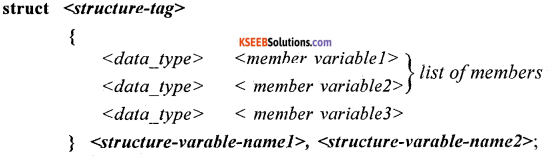is the reserved or keyword used in structure definition.
<structure-tag>: Is the name given by the programmer and used to identify the structure in future re-definitions. They are used to declare structure variables.
<data-type>: Any valid data type in ‘C’
< member variable 1…4>: are names of the variables.
<structure-variable-name 1 ..2>: It is used to access the members of the structure

Example: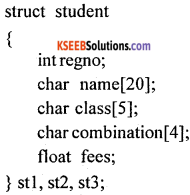PART-D

IV. Answer any seven of the following questions. Each question carries Five marks: (7 × 5 = 35)

Question 27.
Write the features of I generation and II generation of computers.
1. generation computers:1. The first computers used vacuum tubes for electronic circuitry and magnetic drums for memory. They were very expensive to operate and the power consumption was high, generated a lot of heat, which was often the cause of malfunctions.

2. First generation computers were communicated through machine language to perform operations, and they could only solve one problem at a time.

3. Input was based on punched cards and paper tape, and output was displayed on printouts.

2. Second-generation computers: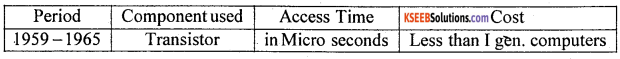1. In the second generation computers, symbolic or assembly languages were used. High-level programming languages were also in use at this time, such as early versions of COBOL and FORTRAN. Their memory consisted of magnetic core technology.

2. Second-generation computers still relied on punched cards for input and printouts for output.Question 28.
Discuss on

1. BCD
2. EBCDIC
3. ASCII

1. Binary Coded Decimal (BCD) code:
In this code each decimal digit is represented by a 4-bit binary number. BCD is a way to express each of the decimal digits with a binary code. In the BCD, with four bits we can represent sixteen numbers (0000 to 1111). But in BCD code only first ten of these are used (0000 to 1001). The remaining six code combinations i.e. 1010 to 1111 are invalid in BCD.

2. EBCDIC:
EBCDIC (Extended Binary Coded Decimal Interchange Code is a binary code for alphabetic and numeric characters that IBM developed for its larger operating systems. In an EBCDIC file, each alphabetic or numeric character is represented with an 8-bit binary number. 256 possible characters (letters of the alphabet, numerals, and special characters) are defined.

3. ASCII code:
ASCII (American Standard Code for Information Interchange) is the most widely used coding system to represent data. ASCII is used on many personal computers and minicomputers. ASCII is a 7-bit code that permits 27 = 128 distinct characters.

The 128 different combinations that can be represented in 7 bits are plenty to allow for all the letters, numbers and special symbols. An eight bit was added. This allowed extra 128 characters to be represented. The extra 128 combinations are used for symbols such as Ç, ü, è, ©, ®, Æ, etc.

Question 29.
Explain the General structure of C++ program with an example.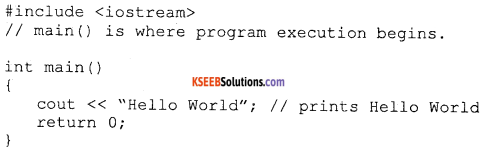The various parts of the above program:

• headers, which contain information that is either necessary or useful to program. For this program, the header is needed.
• The next line // main() is where program execution begins. is a single-line comment available in C++. Single-line comments begin with // and stop at the end of the line.
• The line int main() is the main function where program execution begins.
• The pair of { } indicates the body of the main function.
• The next line cout<< “This is my first C++ program.”; causes the message “This is my first C++ program” to be displayed on the screen.
• The next line return 0; terminates main( )function and causes it to return the value 0 to 1
the calling process.Question 30.
Write the differences between

1. if statement
2. if-else statement with an example for each.

Example: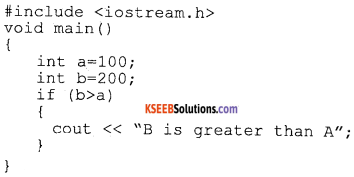Result:
B is greater than A

In the above example the condition in the if statement returns a true value so the text “B is greater than A” is displayed. If value of ‘a’ is greater than value of ‘b’ then no message is displayed.

Example: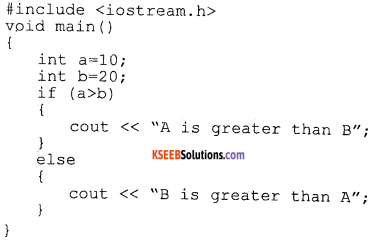Result:
B is greater than A

In the above example the “if condition is true then the message ‘A’ is greater than B” is displayed and in the above program, condition is false so the code in the “else” part is executed.

Question 31.
Illustrate with the help of syntax, differences between While and Do-While looping statements in C++.
The syntax of a while loop in C++ is:

while(condition)
{
statement(s);
}

• Here the condition may be any expression, and true is any nonzero value. The loop iterates while the condition is true.
• When the condition becomes false, program control passes to the line immediately following the loop.
• During the first attempt, when the condition is tested and the result is false, the loop body will be skipped and the first statement after the while loop will be executed.

Syntax of do-while loop

do
{
statements;
} while (condition);

• Here control enters into the body of loop without checking any condition initially and loop iterates while the condition is true at the end.
• When the condition becomes false, program control passes to the line immediately following the loop.
• During the first attempt, no condition is tested at the beginning and checked only at the end and by that time one time the body of loop is executed at least one time.

Question 32.
Explain the accessing elements in two dimensional arrays with example.
Two dimensional array with an example.
Int matrix   = { 91, 96, 90, 94, 99, 95, 87, 92, 98};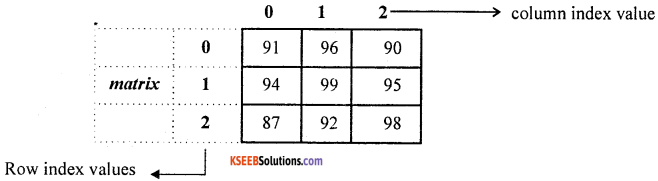Accessing elements:
for (row = 0; row < 3; row++)
for (col = 0; col < 5; col++)
cout << matrix[row] [col];
The above nested looping statements can be used to access all the elements of two dimensional array where variable row and col are used to generate the row and columns index numbers.Question 33.
Write a C++ program to find the area of a triangle given three sides.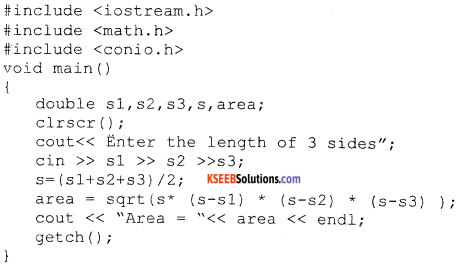Question 34.
Explain the definition of the user-defined function and with the concept of arguments and return value with example.
Functions with argument and with return value:
The calling function gives function call to the called function by passing arguments or values. Then the called function takes those values and performs the calculations and a return a value, that value will be sent back to the calling function. This is done using return statement.

For example: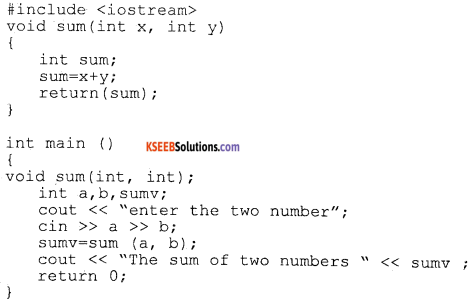In the above example, sum() is a user defined function void sum(int x, int y)having two arguments. The statement int a, b, sumv; declare local variables in main() function. The statement sumv = sum (a, b); is a function call and actual arguments a and b values are sent to formal arguments x and y which is the local variable to sum() function.

The statement return(sum); takes the control back along with the value present in the sum variable and assign it to the sumv variable in the calling function main(). This is how the function with argument and with return value works.Question 35.
What is Mail Merge? Write the steps involved in creating mail merge documents.
The mail merge helps to generate form letters by combining content of one document with content of address. In a situation where a letter is to be sent to address of many persons then mail merge is used. Mail Merge requires the main document and data source document.

1. Main Document:
In Mail Merge, Main Document is the common letter, which contains the common information. It also contains the field names, which contain the instructions for carrying out the merge.

2. Data Source:
Data Source is also called the Data File. It contains information that needs to be inserted in each page of main document. Normally it contains the address information.

3. Form Letter:
Form letter is the resultant document of the mail merge operations, which contains the copy of the main document along with each piece of information stored in the data file.

Question 36.
Explain any five built in functions used in ESS with examples.
The sum(), product() and power() functions are the three mathematical functions, max() and min() functions are the statistical functions.

• The sum() function is used to find the total. For example, = sum(2, 5, 7) then the output is 14.
• The product() function is used to find the multiplication i.e., = product(2, 5) then the output is 10.
• The power function is used to find the exponents i.e., = power(2, 4) then the output is 16.
• The max() function is used to find the maximum values in the series i.e., =max(5, 2, 10, 3, 6), output is 10
• The min() function is used to find the minimum value in the series i.e., =min(5, 2, 10, 3, 6), output is 2.Question 37.
Explain the basic tags in HTML with examples (any five tags).
The basic tags of HTML are <HTML>, <HEAD>, <TITLE>, and <BODY>.
Tags are instructions that are embedded directly into the text of the HTML document. <HTML>